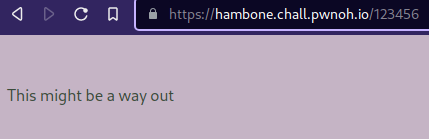# [BUCKEYE] Hambone

I hid the flag somewhere on the website as a 48 byte hex value, so I know you’ll never find it. Just, don’t check out how the background is calculated.

The description hints that the flag will depend on the background color: different paths will make the background color change. Requesting `123456` results in `background: #c7b4c5`Given that the flag path will be an hexadecimal value, we can only insert characters inside the `[0-9a-f]` range.

The attached file shows how the background hexadecimal value is calculated:

``````def get_distances(padded_url : str, flag_path : str):
distances = []
for i in range(3):
# calculate hamming distance on 16 byte subgroups
flag_subgroup = flag_path[i*32:i*32+32]

distances.append(bin(z).count('1'))

return distances
``````

Every 32 charachters of the three subgroups will contribue to one of the three hexadecimal values of the background color. The `get_distance()` function will simply `xor` the value that we insert with the actual flag path and count the number of 1s (i.e. bits that are different).

So we need to bruteforce the flag path by trying values in `[0-9a-f]` for each one of the 96 characters and keeping the ones that maximize the hex background color.

So the final solution:

``````import requests
from bs4 import BeautifulSoup

base_url = "https://hambone.chall.pwnoh.io/"
chars = '0123456789abcdef'

def get_distance(path):
r = requests.get(base_url + path)
soup = BeautifulSoup(r.text)

background = soup.find('body')['style'].split('#')

return background

def get_max_char(path, i):
values = {}

for c in chars:
# insert letter
path = path[:i] + c + path[i+1:]

# store corresponding background hex value
values[c] = get_distance(path)

max_char = max(values, key=values.get)

return max_char, values[max_char]

path = ''

for i in range(32*3):
c, dist = get_max_char(path, i)
path += c
print(f'[+] Path: {path} Dist {dist}')

# get the flag
r = requests.get(base_url+path)
flag = BeautifulSoup(r.text).find('p').text

``````

The final path was

And the flag: `buckeye{th3_b4ckgr0und_i5_n0t_4_l13}`